Maple Flow vs Excel - Making your engineering calculations more productive

# Maple Flow vs Excel

Making your engineering calculations more productive

## Abstract:

Documenting and sharing design calculations is a very important part of engineers duties. Engineers start their careers using Excel for calculations, as a quick means to solve and document basic equations, but after using it for some time, they discover it has limitations when presenting advanced mathematics, often requires workarounds, and can be time consuming to spot errors.

Engineers need easy-to-use calculation software that makes it simple to create and update design worksheets, and many are looking to Maple Flow as an alternative to the spreadsheet format. This paper shows 4 key areas where engineers can improve their productivity and workflow by switching to Maple Flow from Excel.

Maple Flow stands out as calculation software that is aligned to the way engineers approach designs. With Maple Flow, you can capture your thought process, minimize errors that lead to delays, control rising costs, and avoid unexpected outcomes.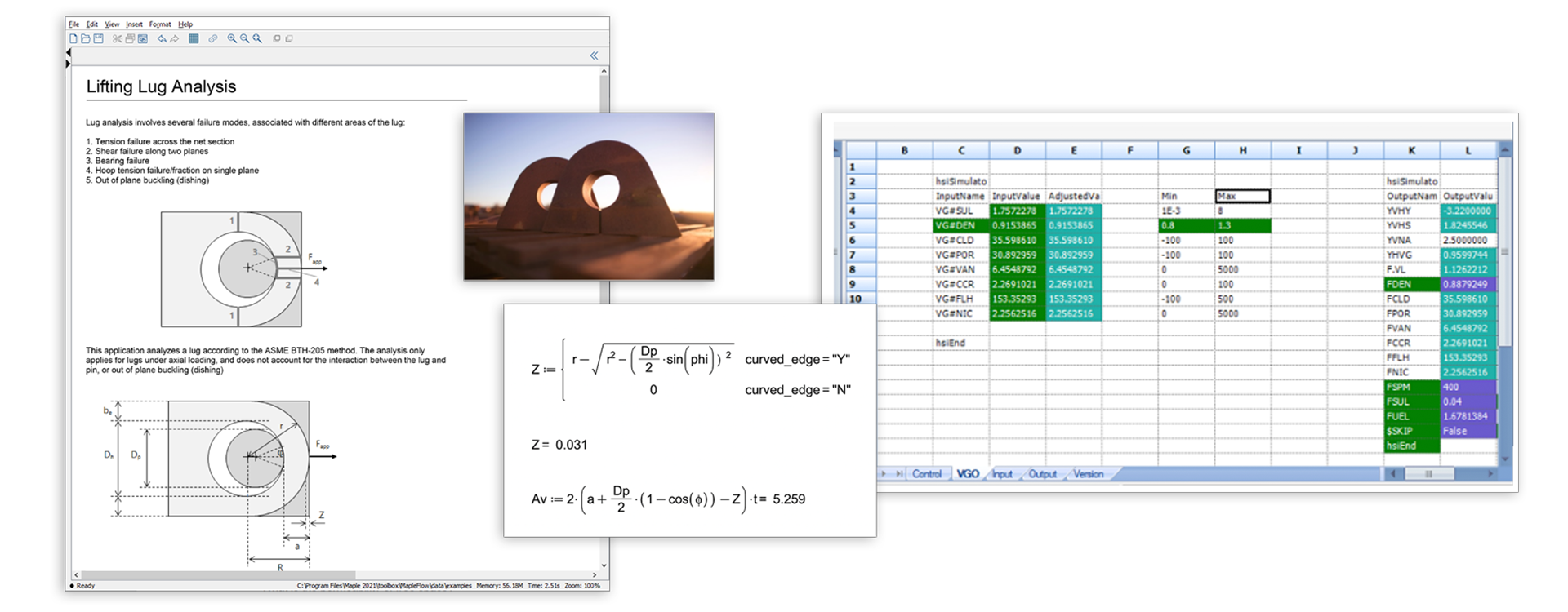Are you using Excel in your engineering projects?

Every engineer starts their career with access to Excel®. It's so easy to open a worksheet and start putting in some values. A quick calculation here, add a multiplier there, change this value because you have new information, and maybe redo the calculation with this number to see what the results would look like. The numbers look good, so you proceed with building a prototype.

Meanwhile, the spreadsheet is shared with another team, where someone adds a few more lines, changes some of the original values, and introduces a number of untraceable errors. A few months down the line, no one knows where any of the figures came from, yet project teams have been basing months of design work on the results of these erroneous calculations.

Cryptic formulas, coupled with a lack of visibility into where data is coming from and how equations are being solved, leave room for errors which result in undesirable and even disastrous consequences.

The high chance of spreadsheet errors

In 2015, research on the risk of errors in business and operational spreadsheets¹ showed that for complex spreadsheets (those with over 100 cells with formulas), the average chance of a particular cell having an error is 3.9%, but this quickly cascades across multiple tabs and pages with the effect that on average 94% of documents studied had at least one mistake.

### Are you using Excel in your engineering projects? Discover a better way.

The report also looked at the types of errors that occurred when working with spreadsheets. In one study, an average 45% of errors were found to relate to the underlying mathematics, such as a mistake in the use of an equation, while 23% of errors were tied to the mechanical way of entering the values in Excel, for example, using the incorrect cell references or copypasting in the wrong place. These errors are made more common by the way Excel formulas are hidden from sight. This shows the importance of checking the contributing calculations as part of a quality document review process.

The fact remains that while Excel has its uses, it is simply not designed for advanced mathematical calculations and their supporting documentation. Engineers and scientists need interactive math systems that enable them to write equations that describe problems using standard mathematical notation, such as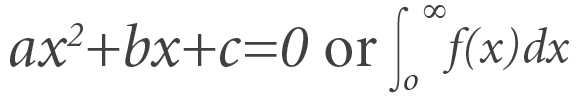and then solve these problems by working with the equations in a natural way.

Maple Flow is one of the best examples of an interactive math system specifically designed for developing, documenting, and solving engineering calculations. Drawing on the advanced Maple math engine, Maple Flow has access to a comprehensive range of solvers that cover all the principal areas of engineering math in a technical document environment that combines text, calculations, images, graphs, and more into a single document. Since Maple Flow is designed for advanced mathematical calculations and allows engineers to capture the design workflow as well as the results, Maple Flow provides many benefits that Excel cannot.

This paper reviews 4 key areas where engineers can improve their productivity and workflow by using Maple Flow instead of Excel.

## 1) Comprehensive Mathematical Capabilities

The ideal tool for engineering projects is one that can handle complex calculations across a broad range of subject areas.

Excel is, at its essence, a business tool, which has evolved to handle some non-business calculations. Its Function Library now includes many math and engineering functions, but can't handle advanced functions such as calculus or provide plots of functions directly. To plot the graph of a function or evaluate points or gradients on a plotted curve, the underlying data must be sorted into tables that can then be used to create plots and graphs. Excel also offers visual basic application (VBA) macros that allow users to create custom functions and automate frequently performed tasks. However, macros work by manipulating spreadsheets, which is not a natural way to approach problem solving.

Maple Flow has over 5000 functions covering virtually every area of mathematics, including calculus, differential equations, statistics, linear algebra, and transforms. It supports numeric calculations as well as highly accurate symbolic calculations. With symbolic calculations, users can evaluate expressions without assigning values to variables. Maple Flow provides tools for large-scale algebraic and numeric problem solving, and it uses the world-leading algorithms from the Maple computation engine to solve problems beyond the reach of any other software system.

Maple Flow also includes a full-featured programming language that can be used to create scripts, programs, and full applications. Designed for mathematical computations, it includes built-in mathematical data structures, operations, and functions specifically for manipulating mathematical objects and equations, making it ideal for advanced engineering calculations.

## 2) Natural Math Notation

Engineers want to describe problems in terms of equations using variables, constants, and operands, and then work through those problems in a logical manner. An effective tool must have the ability to support users in the way they want to work.

Excel does not support standard math notation. An expression like ((B12+2*\$A\$1)/A12)*2.1328 does not represent math in the way engineers express their problems, nor does Excel enable engineers to manipulate equations naturally. There is no flow to how equations are solved, and you have to jump around from cell to cell in order to see where a calculation is performed, and where the result is being used. Excel's equation feature displays equations in a flat format, rather like an image, requiring any updates to be made by hand.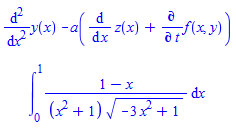Natural math notation helps with presenting and solving differential equations and advanced calculus.

With Maple Flow's free-form canvas, engineers can write equations and formulas in an intuitive and readable manner using standard mathematical notation. Maple Flow then lets you work through your problem in a natural way, with every step clearly visible and well documented. This is particularly helpful when presenting and solving differential equations and using advanced calculus. In Maple Flow, you can see where input values are coming from and where results are being used, allowing you to easily update and manipulate the mathematics in your documents.

Maple Flow enables engineers to solve mathematical problems in much the same way that they would when working them out by hand — albeit much faster, without errors, and with many solvers and analysis tools that far exceed what can be done manually.

## 3) Systems of Units

Engineering calculations involve values that have units — denoting mass, velocity, resistance, density, etc. Tools used for engineering calculations must be robust enough to recognize and correctly handle units in order to perform correct calculations.

Excel is not designed for scientific calculations and does not handle units in an intuitive manner. You cannot perform calculations on numbers in Excel that include units, and any unit reference has to be added separately, taking up a neighboring cell. You can only convert a number in a cell from one unit to another using a function call, such as =CONVERT(C5, "ft", "m").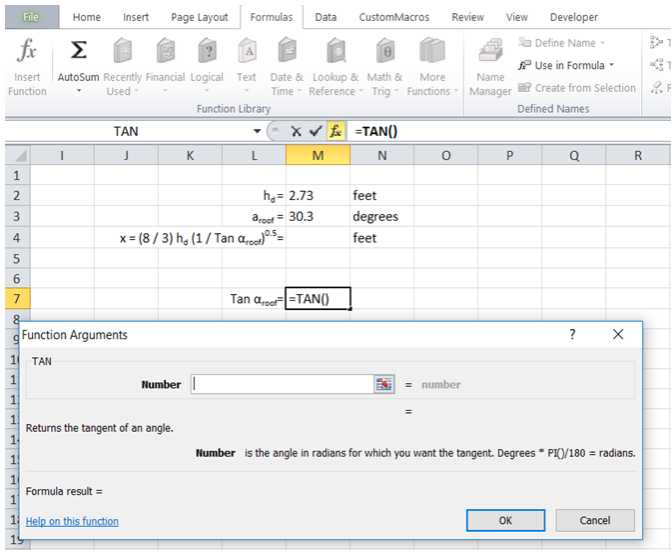Excel defaults to evaluate trigonometric expressions by using radians which can confuse users expecting to enter degrees.

One common area of confusion in Excel is how its default is to evaluate trigonometric expressions like sine, cosine and tangent by using radians, while many structural engineering projects reference values of angles measured in degrees.Maple Flow will allow calculations to use a combination of SI and imperial units.

Maple Flow on the other hand, enables engineers to perform intelligent calculations that include units and it can convert units easily from one unit to another. With a menu of SI and imperial units, it also provides support for the use of units, checking for incompatible choices, and handling the manipulation of units in order to assign the correct unit to a resulting calculation. For example, consider the equation F=ma. In Maple Flow, you can multiply a given mass by an acceleration, and Maple Flow will default the result in Newtons. This is impossible in Excel.

## 4) Review Process for Design Calculations

Engineering calculation sheets are more than the sum of their arithmetic operations. They display properties, assumptions, and requirements, they can be made to show reviewer approvals, and they tie the design back to the requested design outcomes. When created and approved by a Professional Engineer, engineering calculation sheets can also communicate compliance with internationally recognized standards such as Eurocodes, IBC/IRC or JIS.Having a robust review process can verify assumptions and reduce risk for the company.

Having a robust internal review process is crucial to an engineering company's success. It allows engineers to verify assumptions, reproduce calculations, and understand where results come from. Given that clarity and accuracy are vital for advanced engineering calculations, an environment that gives only results is doing just a small part of the job.

Excel is designed primarily for business applications, and so end-results are prioritized while the calculations are hidden. Spreadsheet cells hide formulas and cell references from view, requiring extra steps to check for accuracy. While poor formula syntax is often highlighted by Excel's built-in error checking features, typing in the wrong cell reference by accident won't be highlighted by Excel and remains a serious possibility — one that is time-consuming to find and correct.Formulas and cell references entered in a cell are hidden by default in Excel.

Similarly, Excel does not provide a clean way to add notes and comments into a workflow. Without the option for additional context from annotations, the reader can struggle to determine where inputs are coming from, and why certain values are being used. Excel supports adding comments to a cell, but they are not easily identifiable. The reviewer is forced to hunt for a red triangle in the corner of a cell to know that there is a comment, and then hover over it to read the comment. The comment itself cannot include more than very basic mathematical expressions comprised of characters available on a standard keyboard. It is simply not a good way to convey important project requirements or background information.

Maple Flow is a calculation management platform, and its flexible documentation format provides a rich environment in which to create a complete work history of a project. Your notes, comments, visualizations, and calculations are all retained in a single document. Reviewers can easily see where inputs are coming from, what assumptions have been made, and understand why certain actions were taken. These documents are "live", so if the assumptions change, adjustments can be made to parameters and formulas and the results will re-compute within the original document. The Maple Flow document provides an open audit trail that reduces the risk of errors and costly delays, serving as a record of all project activity.

The Maple Flow platform also helps to retain organizational knowledge. By documenting an entire project's workflow, the knowledge gained over the course of a project is captured in a living document that can be referenced at a future date. This protects the company when employees leave, or when project questions arise about why things were done a particular way.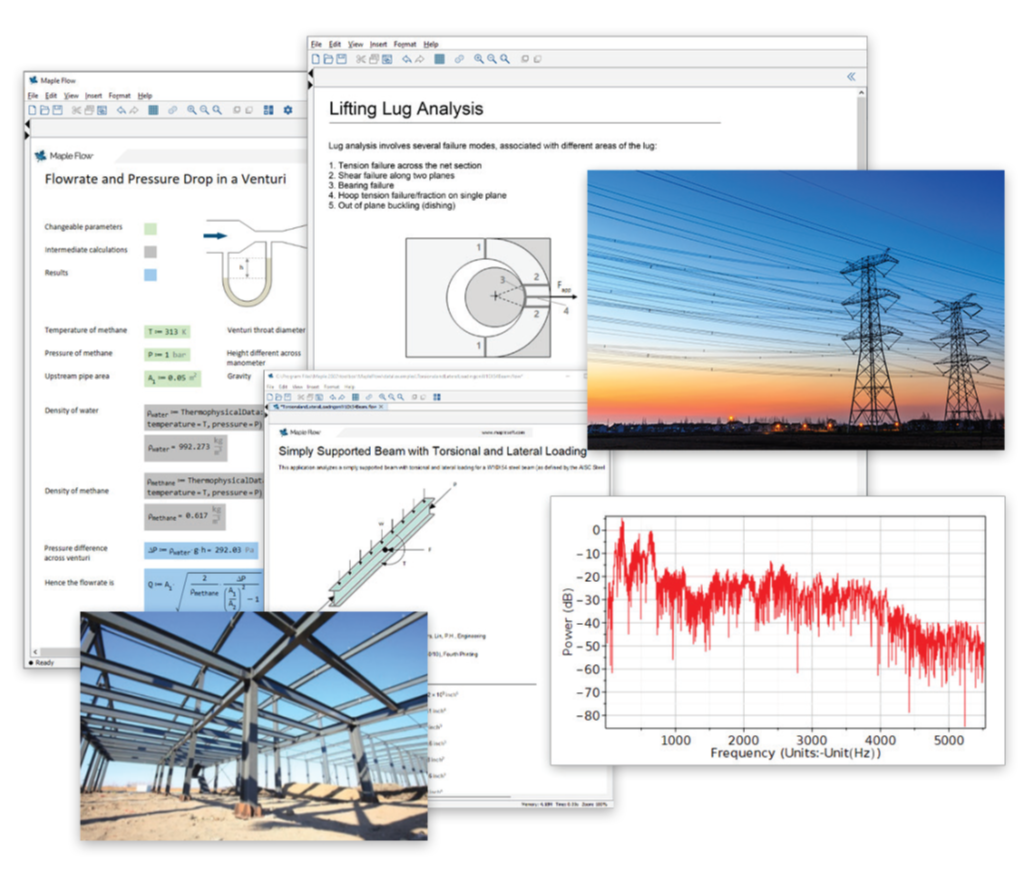Maple Flow allows engineers to document and develop calculations alongside related reference material.

## 5) ConclusionAs the drive for innovation grows, companies are under pressure to deliver better products in less time and at a lower cost. As a result, it is more important than ever that engineers use tools that will get the job done right, the first time. While Excel is good for projects involving a lot of data, it simply cannot handle the scope of mathematical computations required for advanced engineering projects. We're not suggesting you ditch your spreadsheets, but engineering teams also need a robust and powerful interactive mathematical system dedicated to developing and updating design calculations — in other words, engineering teams need Maple Flow.

Maple Flow stands out as calculation software that is aligned to the way engineers approach designs. With Maple Flow, you can capture your thought process, minimize errors that lead to delays, control rising costs, and avoid unexpected outcomes. Just as you wouldn't head out to mow your lawn with a pair of scissors, you shouldn't let the use of inappropriate software jeopardize the success of your engineering projects.